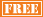January 26
March 9
May 4
June 1

February 9
April 13
June 8

April 20

• ## 2013 GED Test Dates

Varies by State

• ## 2013 AP Exams Test Dates

Calculus AB May 8
Calculus BC May 8
Statistics May 10
Macroeconomics May 16
Microeconomics May 16

# ACT Math

We provide intensive tutoring for students preparing for the mathematics section of the ACT exam. The math section of the ACT Exam includes 60 questions to be completed in 60 minutes. The section consists of questions based on the topics of pre-algebra, elementary algebra, intermediate algebra, coordinate geometry, regular geometry, and trigonometry. The section is scored on a scale of 1 to 36.

We utilize a three phase attack to help students properly prepare for the math section of the ACT Exam. During the course of tutoring sessions, we review numerous simulated ACT type problems covering the math topics for which students are responsible. We teach problem solving strategies and techniques which students can apply to specific types of questions on the exam. And we reinforce ACT syllabus math topics which arise during the review of simulated problems for which students are not sufficiently knowledgeable. These 3 components of our ACT prep program provide students with exam problem recognition the day of the exam and the necessary underlying math foundation and strategies to solve problems efficiently and correctly.

We offer a dynamic, intensive program for students preparing for the math section of the ACT Exam. Our focus and expertise is the math section of the exam. All of our efforts go into the single goal of helping students achieve their highest possible score on the math section of the ACT Exam.

• ## One-on-One ACT Math Program includes:

Initial student consultationInitial student assessmentNumber of sessions determined by student

Review of simulated ACT math problems

Review of strategies for attacking ACT math problems

Review of ACT math syllabus

Homework review following each session

• ## Group ACT Math Program includes:

Initial student consultationInitial student assessmentClass size of no more than 20 students

Review of simulated ACT math problems

Review of strategies for attacking ACT math problems

Review of ACT math syllabus